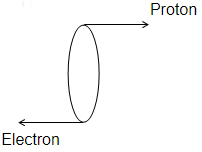In a hydrogen discharge tube it is observed that through a given cross-section 3.13 × 1015 electrons are moving from right to left and 3.12 × 1015 protons are moving from left to right. What is the electric current in the discharge tube and what is its direction

(1) 1 mA towards right

(2) 1 mA towards left

(3) 2 mA towards left

(4) 2 mA towards right

(1)

For any cross-sectionCurrent is opposite to flow of electrons
Let charge on each electron be qe and charge on each proton be qp

Flow of current due to electrons = ne qe    (right)
Flow of current due to protons = npqp       (right)
Total current = neqe+nqqp

$I={n}_{e}{q}_{e}+{n}_{p}{q}_{p}=1mA$ towards right

Direction of current is taken as flow of positive charge.

Difficulty Level:

• 53%
• 30%
• 9%
• 10%